Author: Oscar Cronquist Article last updated on April 19, 2022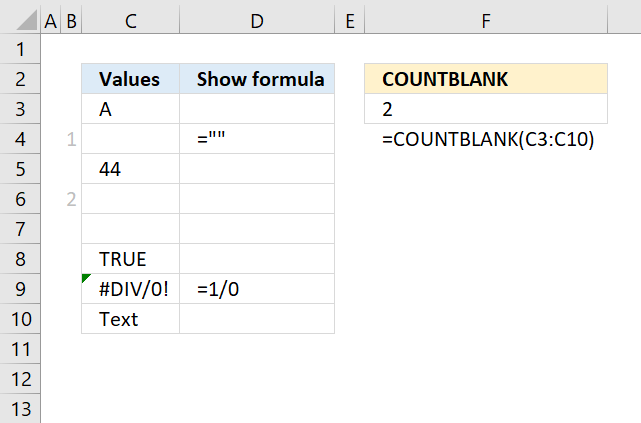The COUNTBLANK function counts empty or blank cells in a range.

The picture above demonstrates the COUNTBLANK function entered in cell F3. The evaluated range is C3:C10, two of the cells contains formulas, three cells seem to be empty but only one is in fact empty.

Cell C4 has a formula that returns a blank, note that the COUNTBLANK function considers this cell empty. Cell C7 has a space character and is therefore counted. Only cell C6 is really empty.

Use the TRIM function to remove space characters.

## 1. COUNTBLANK Function Syntax

COUNTBLANK(value1, [value2], ...)

## 2. COUNTBLANK Function Arguments

 value1 Required. A cell reference to a range for which you want to count empty cells. [value2] Optional. Up to 254 additional arguments like the one above.

The COUNTBLANK function counts errors as not empty.

## 3. COUNTBLANK Function exampleThe formula in cell F3 counts two empty cells in cell range C3:C10. Note, there is also one error value meaning the COUNTBLANK function works with error values as well.

Formula in cell F3:

=COUNTBLANK(C3:C10)

COUNTBLANK(C3:C10)

becomes

COUNTBLANK({"A";"";44;0;" ";TRUE;#DIV/0!;"Text"})

and returns 2. Cell C7 is not empty, it contains a space character. Cell C4 contains a formula, however, the output is nothing. The COUNTBLANK function counts cell C4 as empty.

## 4. Count blanks - arrays and other functions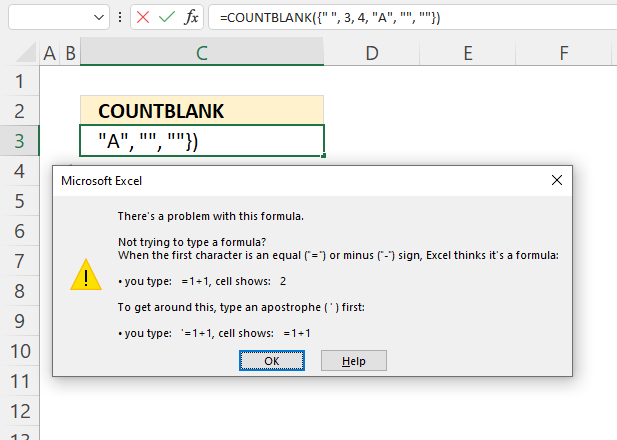This example shows that you can't use hardcoded arrays in the COUNTBLANK function, a dialog box appears telling you there is a problem with the formula.

Formula in cell C3:

=COUNTBLANK({" ", 3, 4, "A", "", ""})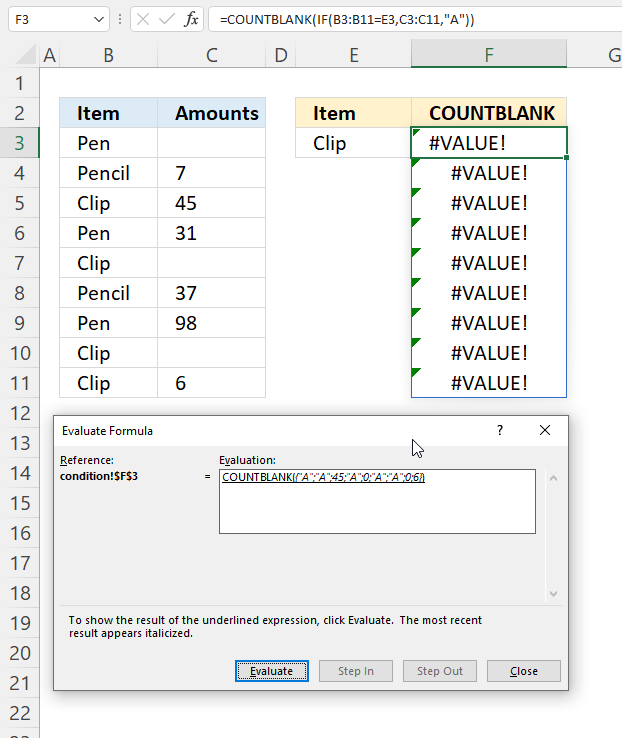You can't use other functions in the COUNTBLANK function, unexpected things may happen.

=COUNTBLANK(IF(B3:B11=E3,C3:C11,"A"))

## 5. Count blanks based on a condition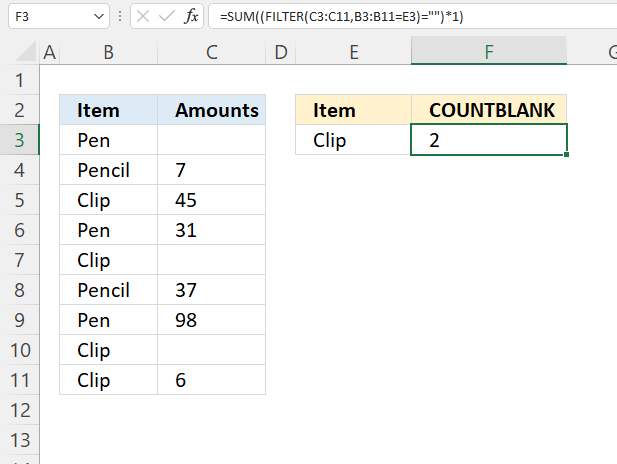Formula in cell C3:

=SUM((FILTER(C3:C11,B3:B11=E3)="")*1)

### Explaining formula

B3:B11=E3

#### Step 2 -

FILTER(C3:C11,B3:B11=E3)

#### Step 3 -

FILTER(C3:C11,B3:B11=E3)=""

#### Step 4 -

(FILTER(C3:C11,B3:B11=E3)="")*1

#### Step 5 -

SUM((FILTER(C3:C11,B3:B11=E3)="")*1)

## 6. Count blanks based on a list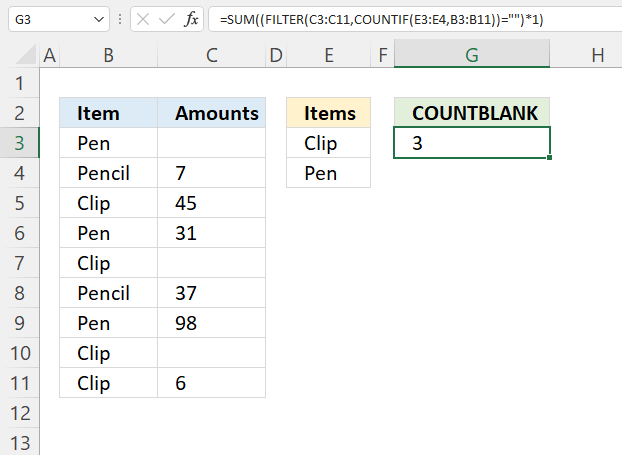Formula in cell C3:

=SUM((FILTER(C3:C11,COUNTIF(E3:E4,B3:B11))="")*1)

### Explaining formula

#### Step 1 -

COUNTIF(E3:E4,B3:B11)

#### Step 2 -

FILTER(C3:C11,COUNTIF(E3:E4,B3:B11))

#### Step 3 -

FILTER(C3:C11,COUNTIF(E3:E4,B3:B11))=""

#### Step 4 -

(FILTER(C3:C11,COUNTIF(E3:E4,B3:B11))="")*1

#### Step 5 -

SUM((FILTER(C3:C11,COUNTIF(E3:E4,B3:B11))="")*1)

## 7. Count blanks in a delimited text string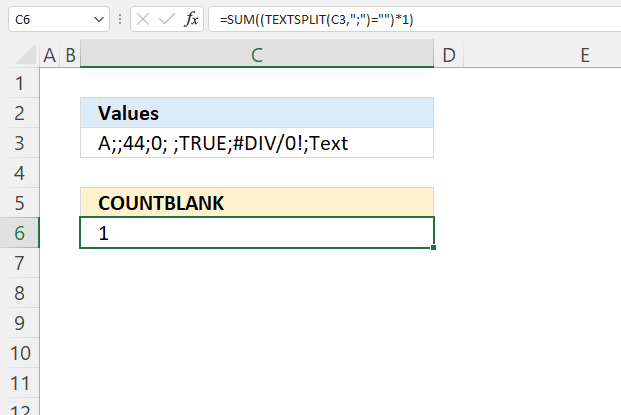Formula in cell C3:

=SUM((TEXTSPLIT(C3,";")="")*1)

### Explaining formula

#### Step 1 -

TEXTSPLIT(C3,";")

#### Step 2 -

TEXTSPLIT(C3,";")=""

#### Step 3 -

(TEXTSPLIT(C3,";")="")*1

#### Step 4 -

SUM((TEXTSPLIT(C3,";")="")*1)

## 8. Count blanks in multiple cell ranges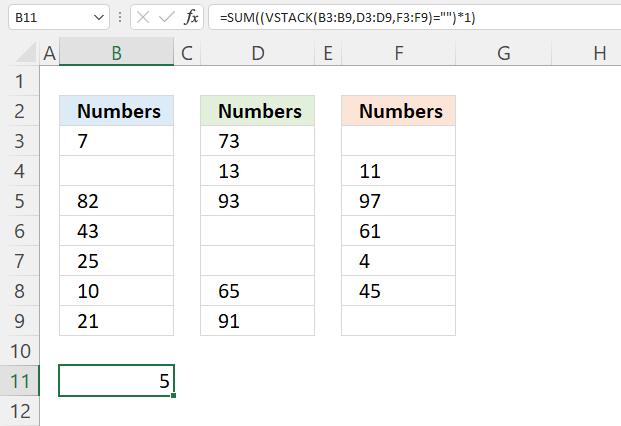Formula in cell B12:

=SUM((VSTACK(B3:B9,D3:D9,F3:F9)="")*1)

### Explaining formula

#### Step 1 - Join arrays

VSTACK(B3:B9,D3:D9,F3:F9)

#### Step 2 - Check if empty

VSTACK(B3:B9,D3:D9,F3:F9)=""

#### Step 3 - Convert boolean values to numbers

(VSTACK(B3:B9,D3:D9,F3:F9)="")*1

#### Step 4 - Calculate a total

SUM((VSTACK(B3:B9,D3:D9,F3:F9)="")*1)